# AC Circuit Theory: What is AC and How its Generated

Published  January 24, 2018   1
E
Author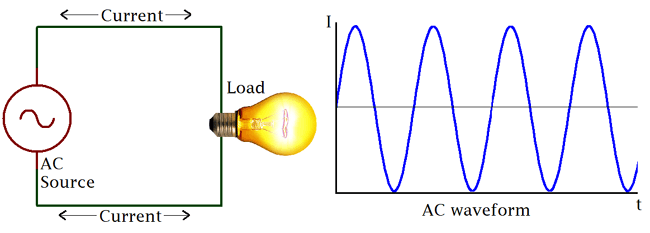### Introduction

An electrical circuit is a complete conductive path through which electrons flow from the source to the load and back to the source. The direction and magnitude of the electrons flow however depend on the kind of source. In Electrical Engineering, there are basically two types of voltage or current (Electrical Energy) source which defines the kind of circuit and they are; Alternating Current (or voltage) and Direct Current.

For the next couple of posts, we will be focusing on the Alternating current, and move through topics ranging from what is Alternating current to AC wave forms and so on.

### AC Circuits

AC circuits as the name (Alternating Current) implies are simply circuits powered by an Alternating Source, either voltage or current. An Alternating Current or Voltage, is one in which the value of either the voltage or the current varies about a particular mean value and reverses direction periodically.

Most present day household and industrial Appliances and systems are powered using alternating current. All DC based plugged in appliances and rechargeable battery based devices technically run on Alternating current as they all use some form of DC power derived from AC for either charging of their batteries or powering of the system.  Thus Alternating current is the form via which power is delivered at the mains.

The Alternating circuit came into being in the 1980s when Tesla decided to solve the long range incapability of the Thomas Edison’s DC generators. He sought a way of transferring electricity at a high voltage and then employ the use of transformers to step it either up or down as may be needed for distribution and was thus able to minimize power loss across a great distance which was the main problem of Direct Current at the time.

### Alternating Current VS Direct Current (AC vs DC)

AC and DC differ in several ways from generation to transmission, and distribution, but for the sake of simplicity, we will keep the comparison to their characteristics for this post.

The major difference between the AC and DC, which is also the cause of their different characteristics, is the direction of flow of electric energy. In DC, Electrons flow steadily in a single direction or forward, while in AC, electrons alternate their direction of flow in periodic intervals. This also leads to alternation in the voltage level as it switches along from positive to negative in line with the current.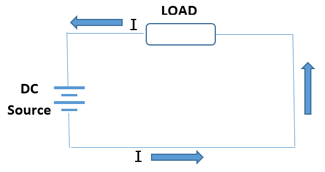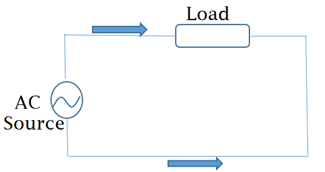Below is a comparison chart to highlight some of the difference between AC and DC. Other differences will be highlighted as we go more into exploring Alternating current Circuits.

 Comparison Basis AC DC Energy Transmission Capacity Travels over long distance with minimal Energy loss Large amount of energy is lost when sent over long distances Generation Basics Rotating a Magnet along a wire. Steady Magnetism along a wire Frequency Usually 50Hz or 60Hz depending on Country Frequency is Zero Direction Reverses direction periodically when flowing through a circuit It steady constant flow in one direction. Current Its Magnitude Vary with time Constant Magnitude Source All forms of AC Generators and Mains Cells, batteries, Conversion from AC Passive Parameters Impedance (RC, RLC, etc) Resistance Only Power Factor Lies between 0&1 Always 1 Waveform Sinusoidal, Trapezoidal, Triangular and Square Straight line, sometimes Pulsating.

### Basic AC Source (Single Coil AC Generator)

The principle around AC generation is simple. If a magnetic field or magnet is rotated along a stationary set of coils (wires) or the rotation of a coil around a stationary magnetic field, an Alternating current is generated using an AC generator(Alternator).

The simplest form of AC generator consists of a loop of wire that is mechanically rotated about an axis while positioned between the north and south poles of a magnet.

Consider the Image below.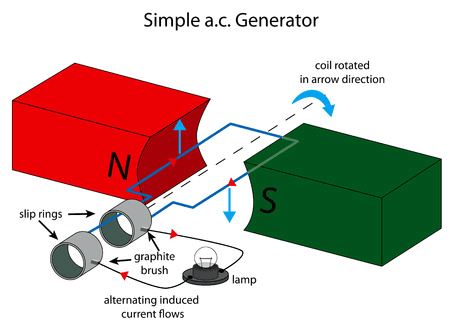As the armature coil rotates within the magnetic field created by the north and south pole magnets, the magnetic flux through the coil changes, and charges are thus forced through the wire, giving rise to an effective voltage or induced voltage. The magnetic flux through the loop is as a result of the angle of the loop relative to the direction of the magnetic field.  Consider the images below;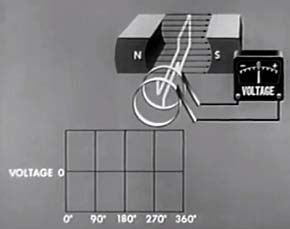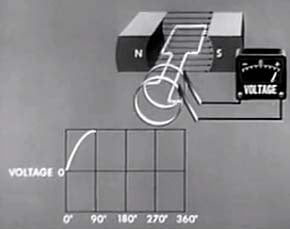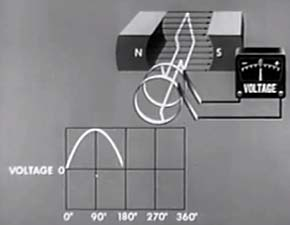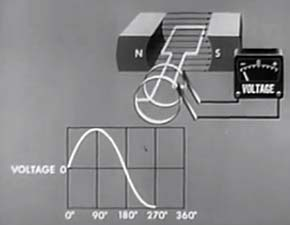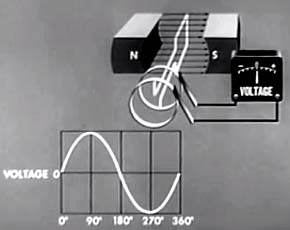From the images shown above, we can deduce that, certain number of magnetic field lines will be cut as the armature rotates, the amount of ‘lines cut’ determines the voltage output. With each change in the angle of rotation and the resultant circular motion of the armature against the magnetic lines, the amount of ‘magnetic lines cut’ also changes, hence the output voltage also change. For instance, the magnetic field lines cut at zero degree is zero which makes the resultant voltage zero, but at 90 degrees, almost all the magnetic field lines are cut, thus maximum voltage in one direction is generated in one direction. The same holds at 270 degrees only that it’s generated in the opposite direction. There is thus a resultant change in the voltage as the armature rotates within the magnetic field leading to the formation of a sinusoidal waveform. The resultant induced voltage is thus sinusoidal, with an angular frequency ω measured in radians per seconds.

The Induced current in the setup above is giving by the equation:

I = V/R

Where V= NABwsin(wt)

Where N = Speed

A = Area

B = Magnetic field

w = Angular frequency.

Real AC generators are obviously more complex than this but they work based on the same principles and laws of electromagnetic induction as described above. Alternating current is also generated using certain kind of transducers and oscillator circuits as found in inverters.

### Transformers

The induction principles on which AC is based is not limited to its generation only but also in its transmission and distribution. As at the time when AC came into reckoning, one of the main issues was the fact that DC couldn’t be transmitted over a long distance, thus one of the main issues, AC had to be solved to become viable, was to be able to safely deliver the high voltages (KVs) generated to consumers who use a voltages in the V range and not KV.  This is one of the reasons why the transformer is described as one of the major enablers of AC and its important to talk about it.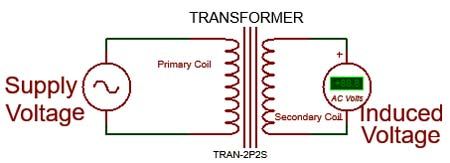In transformers, two coils are wired in such a way that when an Alternating current is applied in one, it induces voltage in the other. Transformers are devices which are used to either step down or step up voltage applied at one end (Primary Coil) to produce a lower or higher voltage respectively at the other end (Secondary Coil) of the transformer.  The Induced voltage in the secondary coil is always equal to the voltage applied at the primary multiplied by the ratio of the number of turns on the secondary coil to the primary coil.

A transformer being a step down or step up transformer is thus dependent on the ratio of number of turns on the secondary coil to the number of turns of conductor on the primary coil. If there are more turns on the primary coil compared to the secondary, the transformer steps down the voltage but if the primary coil has less number of turns compared to the secondary coil, the transformer steps up the voltage applied at the primary.

Transformers has made the distribution of electric power over long range very possible, cost-effective and practical. To reduce losses during transmission, electric power is transmitted from generating stations at high voltage and low current and are then distributed to homes and offices at low voltages and high currents with the aid of transformers.

So we will stop here so as not to overload the article with too much information. In part two of this article, we will be discussing AC waveforms and get into some equations and calculations. Stay tuned.

Submitted by Swapan Kumar Dutta on Sat, 02/10/2018 - 16:36